Publicité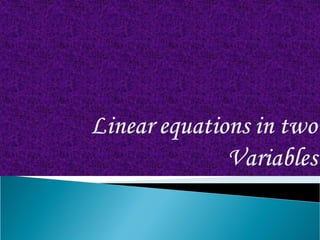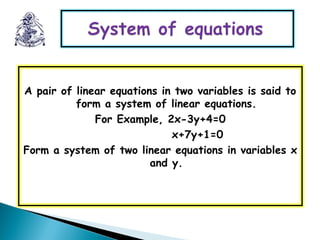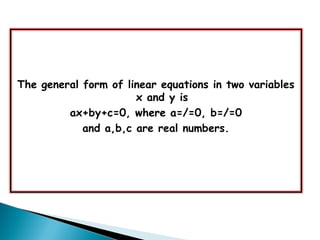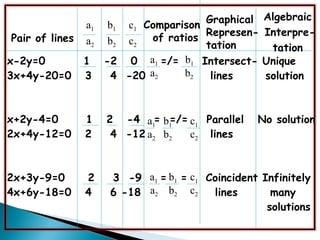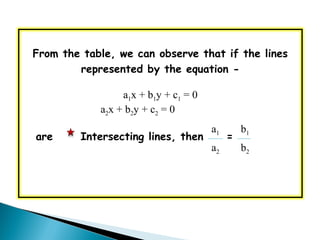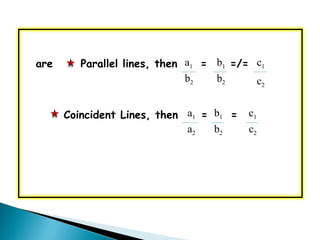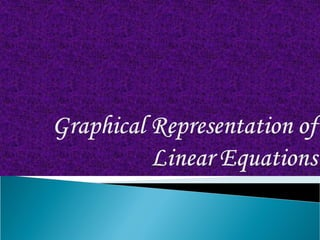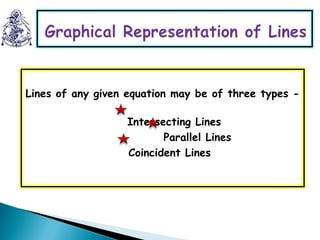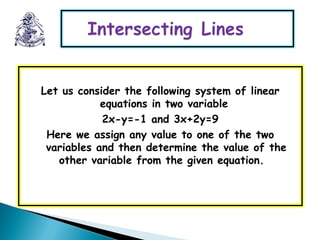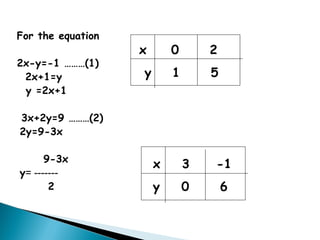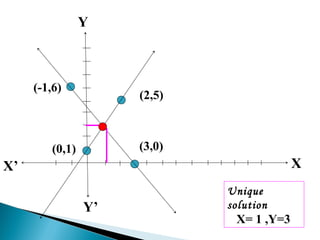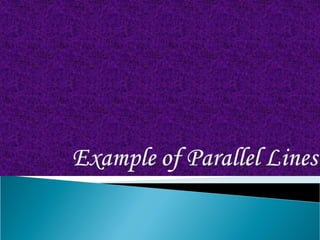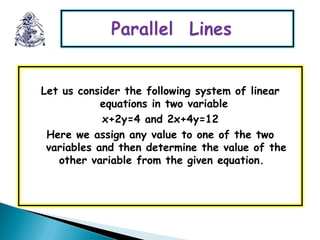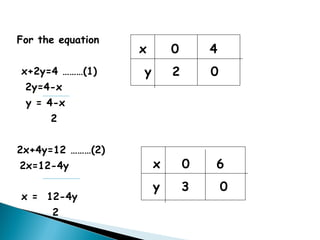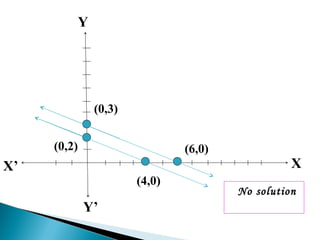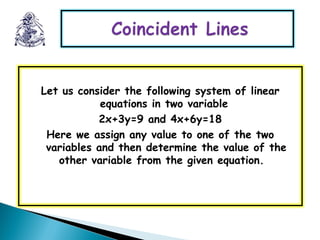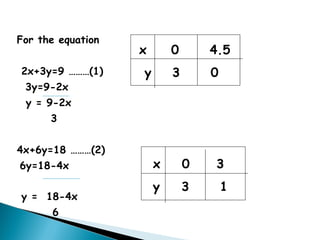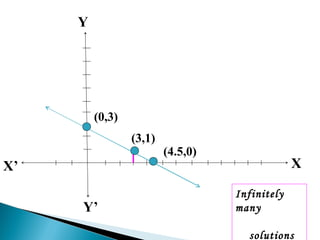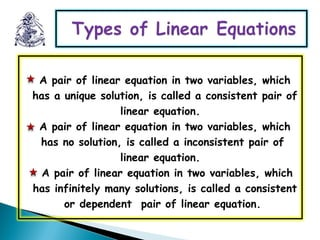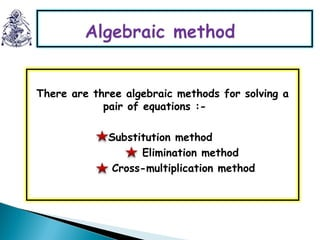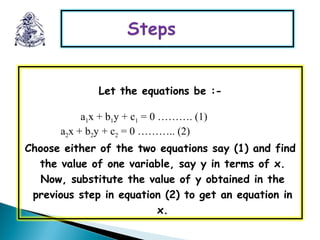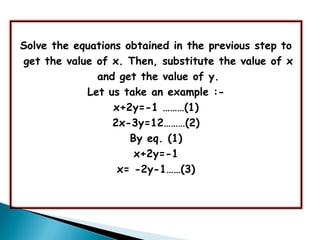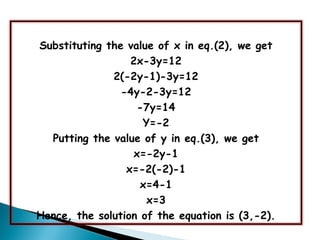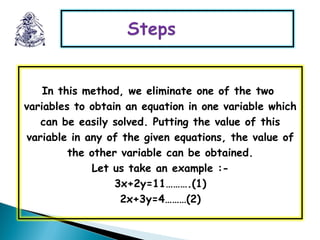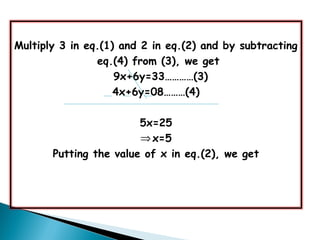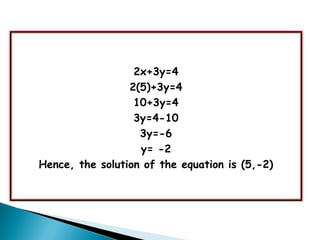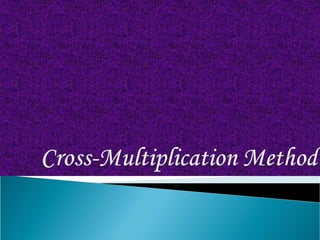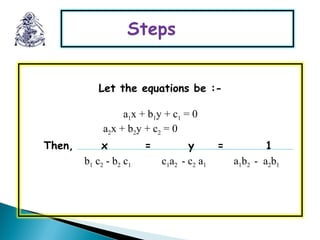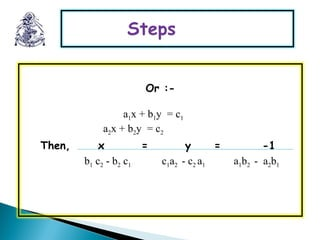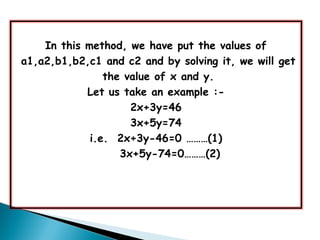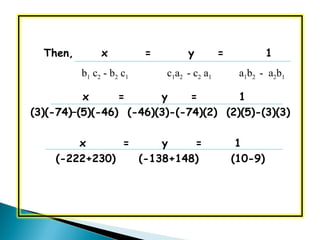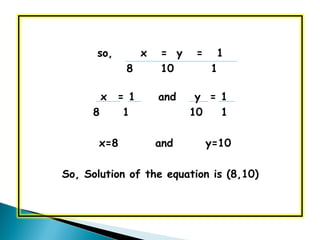1 sur 40
Publicité

### Pair of linear equation in two variables

1. A pair of linear equations in two variables is said to form a system of linear equations. For Example, 2x-3y+4=0 x+7y+1=0 Form a system of two linear equations in variables x and y.
2. The general form of linear equations in two variables x and y is ax+by+c=0, where a=/=0, b=/=0 and a,b,c are real numbers.
3. Pair of lines Comparison of ratios Graphical Represen- tation Algebraic Interpre- tation a1 a2 b1 b2 c2 c1 x-2y=0 1 -2 0 =/= Intersect- Unique 3x+4y-20=0 3 4 -20 lines solution x+2y-4=0 1 2 -4 = =/= Parallel No solution 2x+4y-12=0 2 4 -12 lines 2x+3y-9=0 2 3 -9 = = Coincident Infinitely 4x+6y-18=0 4 6 -18 lines many solutions a1 a2 b1 b2 a1 b1 b1 c1 a1 c1 c2a2 a2 b2 b2 c2
4. From the table, we can observe that if the lines represented by the equation - a1x + b1y + c1 = 0 a2x + b2y + c2 = 0 are Intersecting lines, then = b1 a2 b2 a1
5. are Parallel lines, then = =/= Coincident Lines, then = = b1 c1 a1 c1b1 b2 c2b2 b2 a2 c2 a1
6. Lines of any given equation may be of three types - Intersecting Lines Parallel Lines Coincident Lines
7. Let us consider the following system of linear equations in two variable 2x-y=-1 and 3x+2y=9 Here we assign any value to one of the two variables and then determine the value of the other variable from the given equation.
8. For the equation 2x-y=-1 ………(1) 2x+1=y y =2x+1 3x+2y=9 ………(2) 2y=9-3x 9-3x y= ------- 2 x 0 2 y 1 5 x 3 -1 y 0 6
9. XX’ Y Y’ (2,5) (-1,6) (3,0)(0,1) Unique solution X= 1 ,Y=3
10. Let us consider the following system of linear equations in two variable x+2y=4 and 2x+4y=12 Here we assign any value to one of the two variables and then determine the value of the other variable from the given equation.
11. For the equation x+2y=4 ………(1) 2y=4-x y = 4-x 2 2x+4y=12 ………(2) 2x=12-4y x = 12-4y 2 x 0 4 y 2 0 x 0 6 y 3 0
12. XX’ Y Y’ (0,2) (4,0) (6,0) (0,3) No solution
13. Let us consider the following system of linear equations in two variable 2x+3y=9 and 4x+6y=18 Here we assign any value to one of the two variables and then determine the value of the other variable from the given equation.
14. For the equation 2x+3y=9 ………(1) 3y=9-2x y = 9-2x 3 4x+6y=18 ………(2) 6y=18-4x y = 18-4x 6 x 0 4.5 y 3 0 x 0 3 y 3 1
15. XX’ Y Y’ (0,3) (4.5,0) Infinitely many solutions (3,1)
16. A pair of linear equation in two variables, which has a unique solution, is called a consistent pair of linear equation. A pair of linear equation in two variables, which has no solution, is called a inconsistent pair of linear equation. A pair of linear equation in two variables, which has infinitely many solutions, is called a consistent or dependent pair of linear equation.
17. There are three algebraic methods for solving a pair of equations :- Substitution method Elimination method Cross-multiplication method
18. Let the equations be :- a1x + b1y + c1 = 0 ………. (1) a2x + b2y + c2 = 0 ……….. (2) Choose either of the two equations say (1) and find the value of one variable, say y in terms of x. Now, substitute the value of y obtained in the previous step in equation (2) to get an equation in x.
19. Solve the equations obtained in the previous step to get the value of x. Then, substitute the value of x and get the value of y. Let us take an example :- x+2y=-1 ………(1) 2x-3y=12………(2) By eq. (1) x+2y=-1 x= -2y-1……(3)
20. Substituting the value of x in eq.(2), we get 2x-3y=12 2(-2y-1)-3y=12 -4y-2-3y=12 -7y=14 Y=-2 Putting the value of y in eq.(3), we get x=-2y-1 x=-2(-2)-1 x=4-1 x=3 Hence, the solution of the equation is (3,-2).
21. In this method, we eliminate one of the two variables to obtain an equation in one variable which can be easily solved. Putting the value of this variable in any of the given equations, the value of the other variable can be obtained. Let us take an example :- 3x+2y=11……….(1) 2x+3y=4………(2)
22. Multiply 3 in eq.(1) and 2 in eq.(2) and by subtracting eq.(4) from (3), we get 9x+6y=33…………(3) 4x+6y=08………(4) 5x=25 ⇒x=5 Putting the value of x in eq.(2), we get
23. 2x+3y=4 2(5)+3y=4 10+3y=4 3y=4-10 3y=-6 y= -2 Hence, the solution of the equation is (5,-2)
24. Let the equations be :- a1x + b1y + c1 = 0 a2x + b2y + c2 = 0 Then, x = y = 1 b1 c2 - b2 c1 c1a2 - c2 a1 a1b2 - a2b1
25. Or :- a1x + b1y = c1 a2x + b2y = c2 Then, x = y = -1 b1 c2 - b2 c1 c1a2 - c2 a1 a1b2 - a2b1
26. In this method, we have put the values of a1,a2,b1,b2,c1 and c2 and by solving it, we will get the value of x and y. Let us take an example :- 2x+3y=46 3x+5y=74 i.e. 2x+3y-46=0 ………(1) 3x+5y-74=0………(2)
27. Then, x = y = 1 x = y = 1 (3)(-74)–(5)(-46) (-46)(3)-(-74)(2) (2)(5)-(3)(3) x = y = 1 (-222+230) (-138+148) (10-9) b1 c2 - b2 c1 c1a2 - c2 a1 a1b2 - a2b1
28. so, x = y = 1 8 10 1 x = 1 and y = 1 8 1 10 1 x=8 and y=10 So, Solution of the equation is (8,10)
Publicité